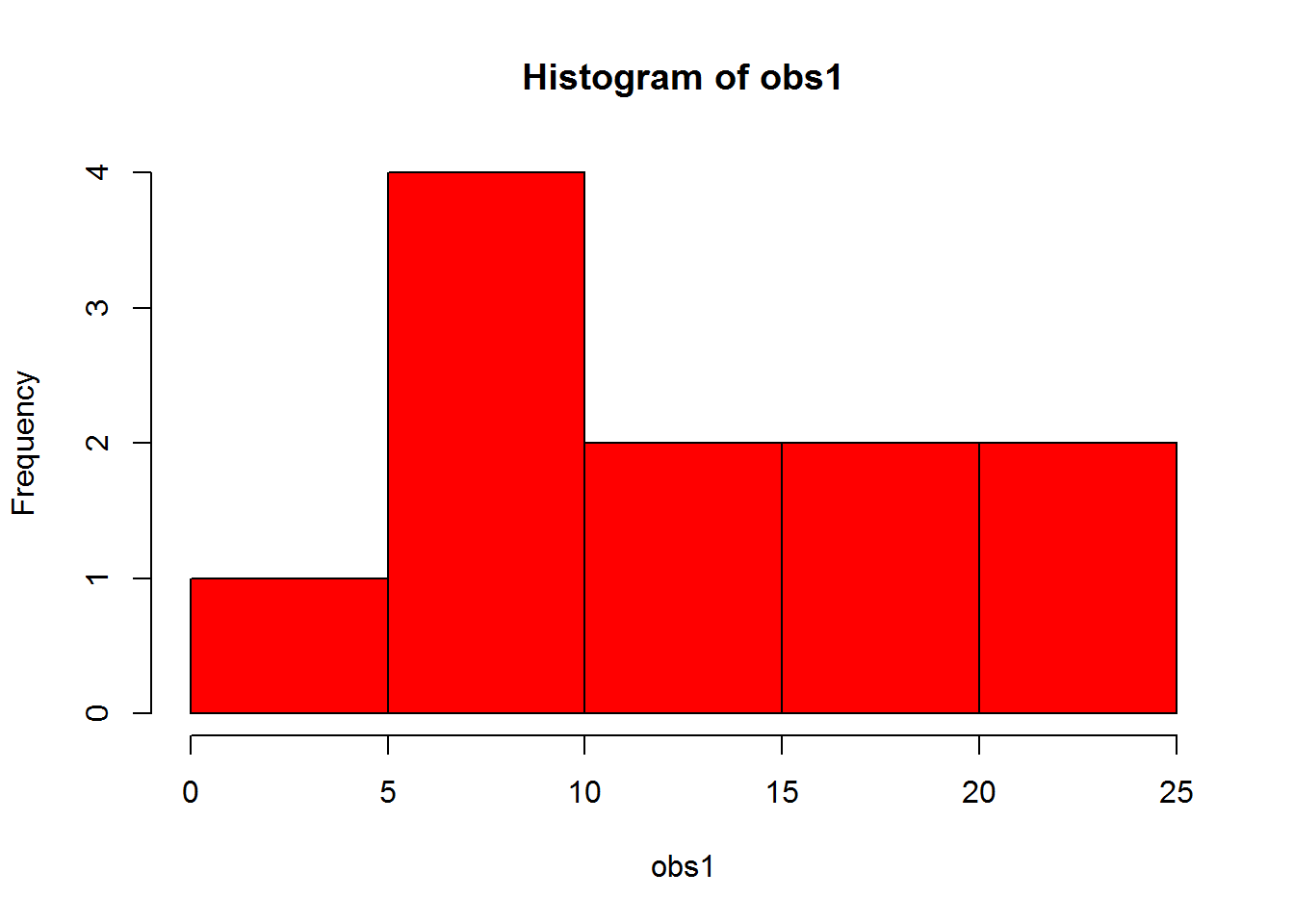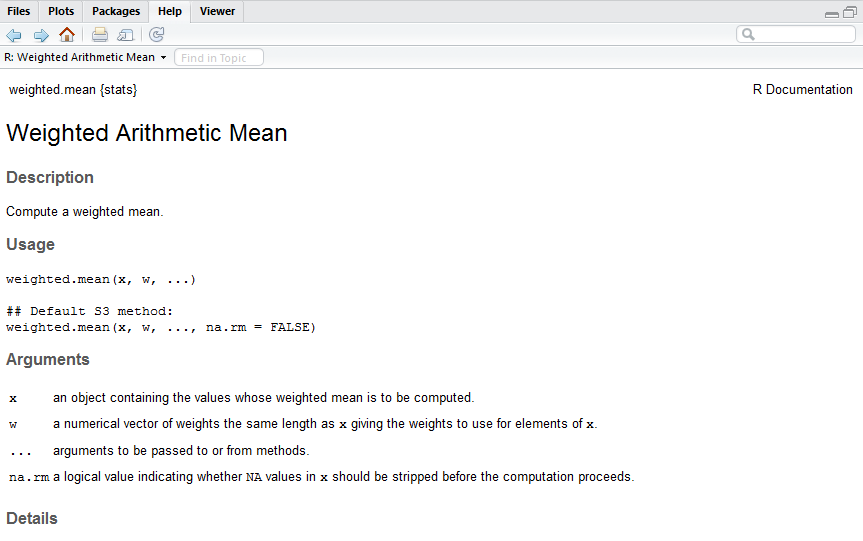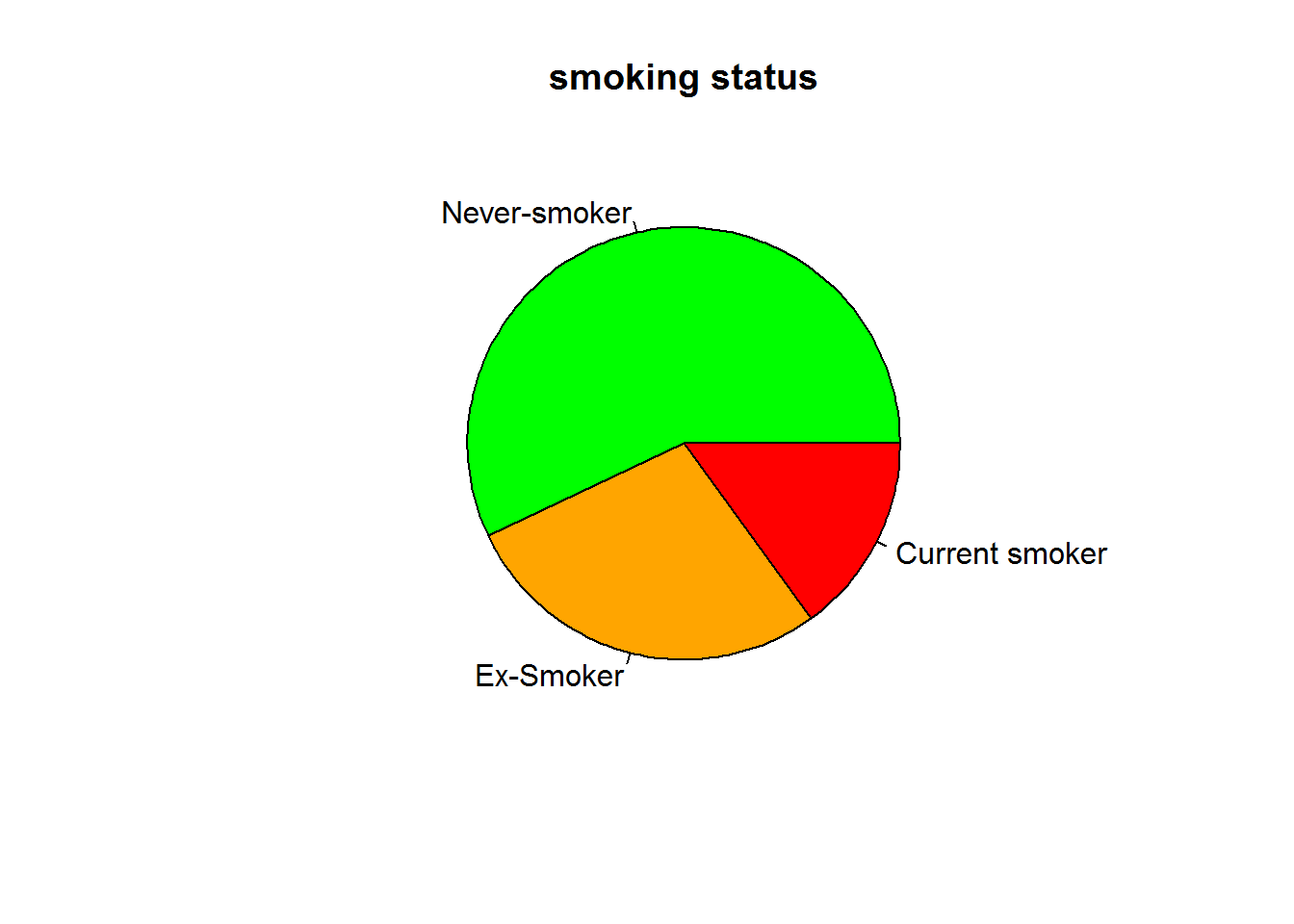## 4. Functions

Section structure:

• 4.1. Changing the color of a histogram
• 4.2. Round the elements of a vector to nearest decimal or integer
• 4.3. Example: analysis of epileptic seizures data
• 4.4. Searching for R-functions
• Exercise 2

At the end of this section, you’ll be able to work with basic R functions.

#### 4.1. Changing the color of a histogram

The functions that we used so far were all of the form function(object). That is, the name of the function is succeeded by the name of the object that is given as input to the function. For most functions, however, additional input can be given to provide additional functionality.

For instance, additional input may be given to hist() to change the color of a histogram. Type and run:

obs1 <- c(4, 10, 23, 13, 16, 20, 21, 14, 8, 9, 9) # Store a set of numeric values as 'obs1'
hist(x = obs1, col = 'red') # Red histogram of obs1To give the histogram a red color we specified an additional argument (or parameter) in the hist() function. To change the color to ‘cornflower blue’, type and run:

hist(x = obs1, col = 'cornflowerblue') # 'Cornflower blue' histogram of obs1

A full list of the default colors in R can be found: here.

#### 4.2. Round the elements of a vector to nearest decimal or integer

Rounding elements of a vector to the nearest integer can be done using the round() function. Type and run:

obs2 <- c(1.332430, 5.668487, 2.333425) # Store a set of numeric values as 'obs2'
obs2int <- round(x = obs2) # Round the values of obs2 to the nearest integer
obs2int

To round to the nearest third decimal you use the digits argument. Type and run:

obs2dec <- round(x = obs2, digits = 3) # Round the values of obs2 to nearest three digits
obs2dec

But be careful with rounding when you want to use these data for later analysis! To illustrate, type and run:

mean(x = obs2) # The mean of the original obs2 vector
mean(x = obs2int) # The mean of the obs2 after rounding to nearest integer

You can see that the rounding of the data has changed the estimate of the mean. Indeed, there are only a few situations where rounding of data is a good idea! It is typically much more sensible to round the results of your analysis. For instance by:

round(x = mean(obs2), digits = 2) # mean of obs2 rounded to the nearest two digits

#### 4.3. Example: analysis of epileptic seizures data

Consider the following data on 31 patients suffering from epilepsy in the first two weeks after starting a drug treatment to prevent epileptic seizures (for those of you who are interested: the drug was called Progabide):

Number of seizures: 0 1 2 3 4 5 6 8 10 11 18 19 22 102
Number of patients: 3 4 5 3 3 2 2 2 1 2 1 1 1 1

The data are displayed in a frequency table format. It can be read as: 3 patients suffered 0 seizures within first two weeks, 4 patients suffered 1 seizure within first two weeks, etc. The source of data can be found here (see p. 44).

To generate two vectors, one containing the number of seizures and the other containing the number of patients, type and run:

num.seiz <- c(0, 1, 2, 3, 4, 5, 6, 8, 10, 11, 18, 19, 22, 102) # Store the number of seizures 'num.seiz'
num.pat <- c(3, 4, 5, 3, 3, 2, 2, 2, 1, 2, 1, 1, 1, 1) # Store the number of observations (i.e. patients)

Now, suppose we are interested in the average number of seizures suffered by the patients in this period. The first function that may come to mind is probably the mean() function. However, applying mean(x = num.seiz) would give the wrong result for these data! Instead, we need to use the function weighted.mean().

For more information on how to use the weighted.mean() function, you can consult the function manual. Type and run:

?weighted.mean # See the manual of weighted.mean

The manual (or “help file”) for the function weighted.mean is now displayed in the lower right panel:The weighted.mean() function allows specification of three arguments: x, w, and na.rm ( ... can be ignored for now). The manual shows arguments xand w are used to indicate the observations (‘values’) and the weights for the observations, respectively. The na.rm argument (short for ‘remove values that are not available’) is used to indicate how the function should deal with missing values1 in the data, which is not an issue for the current analysis.

To use the weighed.mean function for our analysis, type and run:

weighted.mean(x = num.seiz, w = num.pat) # Determine the weighted average
 8.580645

Note that this can be simplified to weighted.mean(num.seiz, num.pat) as long as the order of the arguments is unaffected. However, to avoid mistakes, we encourage the explicit use of the argument name whenever more than one argument is specified.

#### 4.4. Searching for R-functions

So far we have given you the function names to perform the required analysis. You may now wonder: how do I find out which function(s) I can use to perform my own analysis?

There are a variety of ways to get you to the appropriate function(s):

• See also. To search for related function in the help file of a function you are already familiar with. For instance, type and run ?mean. Under See also you’ll find links to the helpfiles of the related functions weighted.mean, mean.POSIXct and colMeans.
• help.start(). If you run this command a new window will be opened in the helper viewer with several helpful links. For instance: you can use Search Engine & Keywords to search for the appropriate function for your analysis.
• Rseek.org. An R search engine.
• Cheat sheets’. There are many cheat sheets that you find online. For instance: this one.

In our experience, however, a simple Google search is often quicker and sufficient. Suppose that we want to describe the variability of observations in a numeric vector by the interquartile range. A Google search will return multiple websites from which you will quickly learn that the function you are looking for is IQR(). Type and run:

obs3 <- c(1.1, 2, 1.4, 1.9, 2.5, 3.7, 4.2) # Store a set of numeric values as 'obs3'
IQR(obs3) # Determine the interquartile range
 1.45

Notice that R is case sensitive! This means that IQR(), Iqr() and iqr() are considered different function names in R (the latter two do not exist).

Finally, there is no such thing as “the correct R function”. There are many functions with overlapping functionality.

#### Exercise 2

Consider the hypothetical data on smoking status for 100 individuals: 57 subjects never smoked, 28 subjects qualify as ex-smokers, and 15 are current smokers. These data can be visualized by means of a so-called pie chart:Try to replicate this chart. Feel free to use any online information that you can find.

The solution to this exercise can be found here.

1. In case you’re wondering: In R, missing values are represented by NA. For instance, when we have three observations, one of which is missing, we could specify e.g. obs4 <- c(21.3, NA, 25)`.